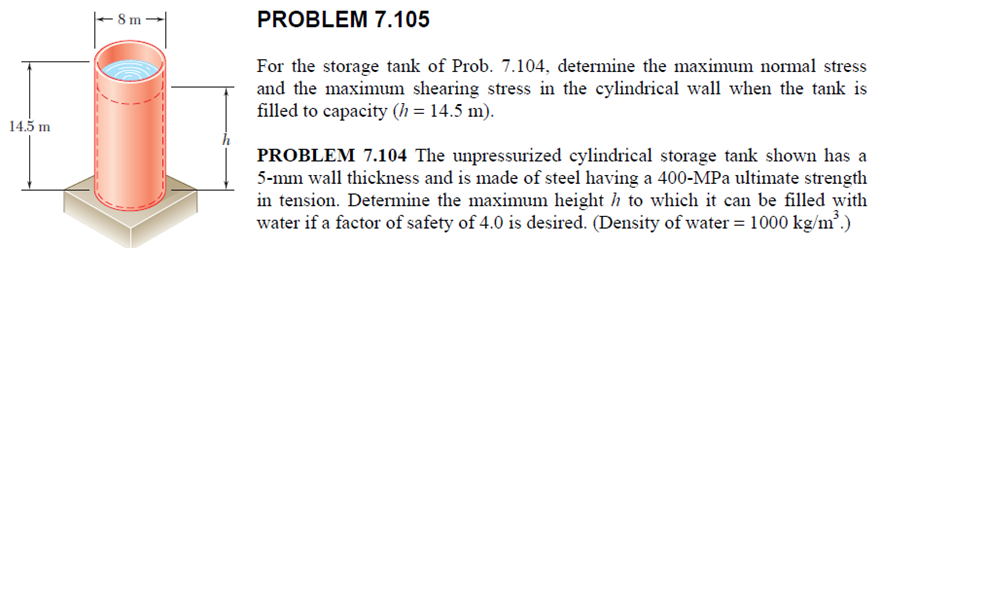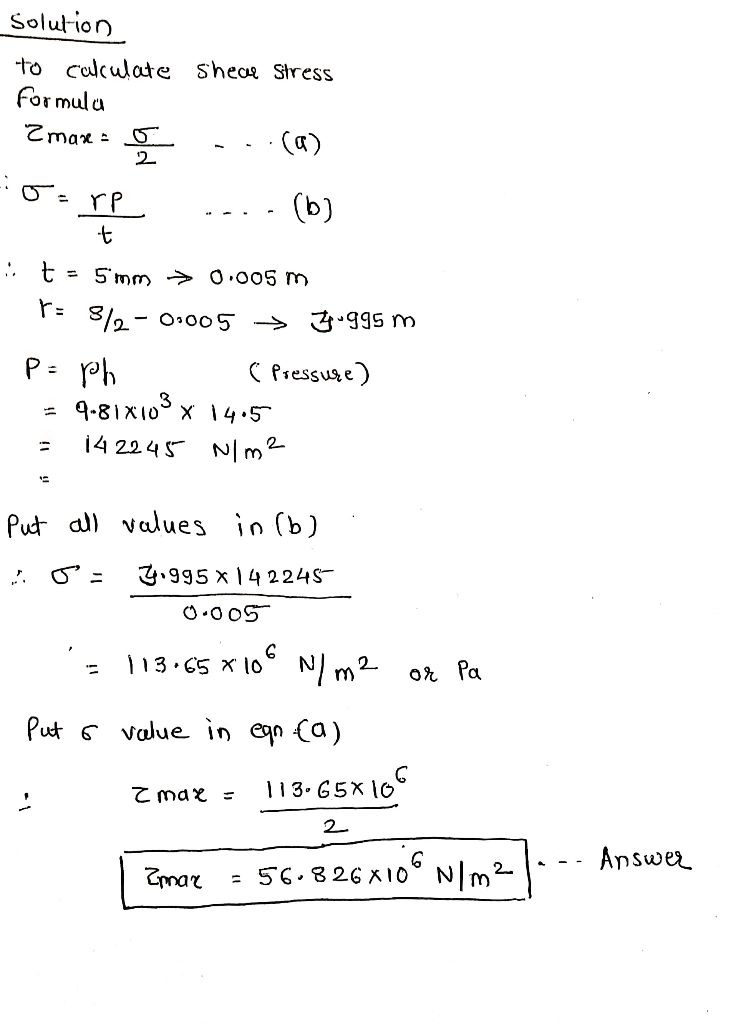Homework Help Question & Answers

PROBLEM 7.105 8 m For the storage tank of Prob. 7.104, determine the maximum normal stress and the maximum shearing stress in the cylindrical wall when the tank is filled to capacity (h 14.5 m). 14.5...PROBLEM 7.105 8 m For the storage tank of Prob. 7.104, determine the maximum normal stress and the maximum shearing stress in the cylindrical wall when the tank is filled to capacity (h 14.5 m). 14.5 m PROBLEM 7.104 The unpressurized cylindrical storage tank shown has a 5-mm wall thickness and is made of steel having a 400-MPa ultimate strength in tension. Determine the maximum height h to which it can be filled witlh water if a factor of safety of 4.0 is desired. (Density of water 1000 kg/m)Add Answer of: PROBLEM 7.105 8 m For the storage tank of Prob. 7.104, determine the maximum normal stress and the maximum shearing stress in the cylindrical wall when the tank is filled to capacity (h 14.5 m). 14.5...
More Homework Help Questions Additional questions in this topic.

• 33% 1. For the stress elements (shown in figures la and lb). Using Mohr's circle determine: The principal stresses σ1 and σ2 and the maximum in-plane shear stress-max and the a) normal stress on...

Need Online Homework Help?

Get FREE EXPERT Answers
WITHIN MINUTES
Related Questions